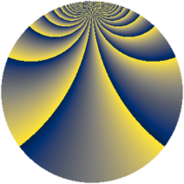# Properties

 Label 1815.2.bhLevel $1815$ Weight $2$ Character orbit 1815.bh Rep. character $\chi_{1815}(23,\cdot)$ Character field $\Q(\zeta_{44})$ Dimension $5200$ Sturm bound $528$

# Related objects

## Defining parameters

 Level: $$N$$ $$=$$ $$1815 = 3 \cdot 5 \cdot 11^{2}$$ Weight: $$k$$ $$=$$ $$2$$ Character orbit: $$[\chi]$$ $$=$$ 1815.bh (of order $$44$$ and degree $$20$$) Character conductor: $$\operatorname{cond}(\chi)$$ $$=$$ $$1815$$ Character field: $$\Q(\zeta_{44})$$ Sturm bound: $$528$$

## Dimensions

The following table gives the dimensions of various subspaces of $$M_{2}(1815, [\chi])$$.

Total New Old
Modular forms 5360 5360 0
Cusp forms 5200 5200 0
Eisenstein series 160 160 0

## Trace form

 $$5200q - 40q^{3} - 36q^{6} - 44q^{7} + O(q^{10})$$ $$5200q - 40q^{3} - 36q^{6} - 44q^{7} - 88q^{10} + 28q^{12} - 72q^{13} - 18q^{15} + 424q^{16} - 54q^{18} - 28q^{21} + 44q^{22} - 20q^{25} - 40q^{27} - 76q^{28} - 38q^{30} - 56q^{31} + 42q^{33} + 108q^{36} - 20q^{37} - 96q^{40} - 24q^{42} - 44q^{43} - 140q^{45} - 88q^{46} - 104q^{48} + 28q^{51} - 132q^{52} - 232q^{55} + 4q^{57} - 76q^{58} + 50q^{60} - 88q^{61} + 18q^{63} - 44q^{66} + 12q^{67} + 68q^{70} - 246q^{72} - 44q^{73} + 14q^{75} - 184q^{76} + 84q^{78} - 56q^{81} - 68q^{82} - 36q^{85} - 104q^{87} - 20q^{88} + 192q^{90} + 224q^{91} - 128q^{93} - 172q^{96} - 108q^{97} + O(q^{100})$$

## Decomposition of $$S_{2}^{\mathrm{new}}(1815, [\chi])$$ into newform subspaces

The newforms in this space have not yet been added to the LMFDB.